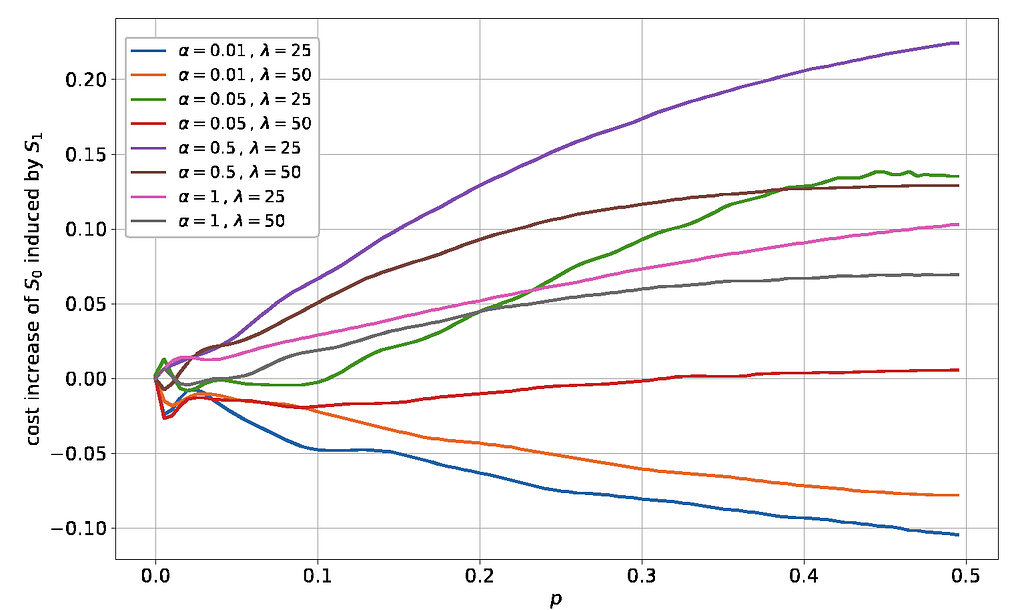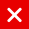# Equilibria in the Tangle: let me try to explain… (part 2)

In our previous post, we explained the importance to find a Nash Equilibrium for the game of attaching transactions to the IOTA Tangle. In the paper (Equilibria in the tangle) we proved its existence and showed that, on this point, all the selfish nodes would choose a similar strategy. However, we still need to see how this Nash Equilibrium looks like. While the theory proves the existence of an “almost symmetric” Nash Equilibrium, this result does not say anything about the strategy nature and which effect it would have on the overall functioning of the tangle. Due to the complexity of the system, we must turn to computer simulations in order to analyze these points.

Over the course of a couple weeks, simulations of the tangle were run on an Intel Xeon 12 core 3.20 Ghz processor, testing samples of approximately 50,000 transactions, varying parameters related to the arrival rate of new transactions λ, the cost (a variable similar to the average time needed for a transaction to be confirmed) as defined in the paper, the “randomness” α of the default random walk tip selection algorithm, the network propagation delay h, and the percentage γ of nodes behaving selfishly. Through these simulations, we were able to empirically find equilibria for the particular kinds of strategies tested and we showed that, even when a large fraction of nodes choose a greedy tip selection strategy, they do not have too much to gain by being selfish. Additionally, we showed that the non-selfish nodes, using the default tip selection strategy, will not lose efficiency significantly by the presence of the greedy nodes.

To begin with, assume that the proportion of selfish nodes which will be able to choose between the default tip selection strategy (S₀ in the paper) and a particular “optimized” tip selection strategy (S₁ in the paper) is fixed and given by γ. The rest of the nodes will not be able to choose their strategy, so they will always use strategy S₀. Such mixed strategy game will be equivalent to a second game where a portion θ of the greedy nodes will choose strategy S₁ and the rest of the selfish nodes will choose strategy S₀. Just for simplification, from now on, we are considering this alternative game. Then, we ask: of these selfish nodes, how many will choose the default tip selection strategy under their own volition? Well, that fraction will of course be at the point where the cost of choosing the default strategy is equal to the cost of choosing the optimized one– i.e. the point where no selfish player has anything to gain by deviating from his own current strategy. We call this point the Nash Equilibrium.

For a given system (that means, for given λ/h and α) the costs of the nodes will only depend on p, the percentage of issued transactions under the strategy S₁(note that, if we have a percentage γ of selfish nodes in the system, and a percentage θ of these nodes choose strategy S₁, we will have p= γθ). So, when we talk about finding Nash equilibria, even though we are talking about values of θ -that is, the variable related to the greedy nodes strategy- we have to explore and study how the costs will vary on p. Then, we “translate” this percentage p to the variable θ.

There are three possible outcomes:

1. The default strategy is always better, for all values of possible p. In this case, all the selfish nodes will choose the default strategy (that means, θ=0).
2. The ‘optimized’ strategy is always better, for all values of possible p. All the selfish nodes will choose the “optimized” strategy (that means, θ=1).
3. If none of the 2 options above holds true, the default strategy will equal the “optimized” strategy at a particular point p. At this p (actually, at θ related to this p), the Nash equilibrium of the system will be found.

For the following graphics (Figure 1), the default tip selection strategy used in the simulation had a high degree of randomness (α = 0.01). The simulations were run for two incoming transaction rates (λ = 25 and λ = 50). Now we ask: which one of the three possible scenarios above will be true? Do the selfish nodes always choose the default strategy? Or do they always choose the “optimized” strategy? Or do only a proportion of these nodes choose one strategy over the other? In the depicted cases, the cost of the default strategy intersects with the cost of the “optimized” strategy. The proportion of nodes using the strategy S₁ in this intersection point is roughly p=0.07 for λ=25 and p=0.12 for λ=50. These percentages p now must be translated to θ, where will be the Nash Equilibrium points. To do that, we will analyze the data for λ=25, using two distinct examples, that will lead us to the two possible outcomes of this problem.Figure 1: S₀ is the default strategy and S₁ is the “optimized” strategy. p is the proportion of selfish nodes choosing the optimized strategy over the default one. The Nash Equilibrium is where the lines (for the two respective transaction rates λ) intersect.

1. Suppose that we have a percentage γ=0.5 of nodes behaving selfishly. Which is the maximum possible percentage of issued transactions under the strategy S₁? Clearly, it is 0.5, since the non-greedy nodes can not choose the strategy S₁. So, the possible p will be 0≤p≤0.5. Now, in order to obtain a percentage of issued transactions under the strategy S₁ of p=0.07, we must have θ=0.14. So, this will be the Nash Equilibrium.

2. Now suppose that we have a percentage γ=0.05 of nodes behaving selfishly. Which is the maximum percentage of issued transactions under the strategy S₁? Clearly, it is 0.05, for the same reasons of the other example. So, the possible p will be 0≤p≤0.05, meaning that, in this case, we will always have an ‘optimized’ strategy with a smaller cost, for all values of possible p. So, all the selfish nodes will choose the ‘optimized’ strategy (that means, θ=1).

Summing up, if the costs of the nodes under strategy S₁ equals the costs of the nodes under strategy S₀ at p=p , the Nash equilibrium will be at θ = min(1,p/γ). One possible direct application of this information could be for the improvement (in a game theoretical aspect) of the default tip selection algorithm. If we change the current default tip selection algorithm for one that uses S₁ at a percentage p of the time and S₀ the rest of it, since the selfish nodes will also tend to that point when on equilibrium, the selfish nodes will have no incentive at all to deviate from the default strategy.

Now that we have already found the Nash equilibrium, a question remains unanswered: what cost does the presence of selfish strategies imposes on the overall functioning of the system? Will the non-selfish nodes be harmed (when compared to a situation where there are no selfish nodes at all) in a meaningful way?

The following graphic (Figure 2) shows the average cost increase imposed on the nodes following the default strategy by the selfish nodes. Let W(p) be the non-greedy nodes costs depicted in Figure 1. The cost increase is calculated as (W(p)-W(0))/W(0), so the cost increase is the difference (in percentage) of the cost of a non-selfish node in the presence of a percentage p of selfish transactions and the cost of a non-selfish node when there are no selfish transactions at all. As shown in the graph, the average cost increase imposed on the non-greedy nodes found is actually negative, meaning that the presence of selfish nodes, on average, will not harm the other nodes for the low α regime at all.Figure 2 — Non-selfish nodes cost increase induced by the presence of selfish nodes, for several α and λ

Note that the above result will not necessarily hold for a higher α. Also, we are not claiming here that the selfish nodes always help the non-selfish ones, since we are just analyzing averages, not all the distribution of the node’s costs. For instance, wide distributions (meaning that some nodes will have a very low cost and the others a very high one) might disturb the system functioning. So, a deeper analysis should be done on this subject in further studies.

Moreover, we show that the gains of the greedy nodes over the non-greedy ones are not economically attractive (note in Figure 3 that the maximum possible gain in this case is a little over 10%) when compared with some intrinsic growth in the computational costs, meaning that the gains from acting selfishly are small (or perhaps even negative) when compared to simply following the default tip selection algorithm of the non-selfish nodes. Also, we don’t have in consideration the “social costs” of being greedy, since, when a node is spotted as selfish, it can be avoided by the other nodes, which means that, in the long run, this node may be “banned” from the network, even when nobody is forcing the other nodes to do so.Figure 3: Gains of the greedy strategy S₁ over the default strategy S₀, in percentage, for α = 0.01. The Nash Equilibrium is where δ = 0.

Summing up, the preliminary results of this research have confirmed some of the intuitions of the authors about how the Tangle might evolve in the presence of greedy nodes. Further research will include the expansion of the space of possible greedy tip selection strategies, running simulations with ML and AI techniques, and continue to optimize the default tip selection algorithm to be the optimal strategy for both selfish and non-selfish nodes.

You might also like

This website uses cookies to improve your experience. We'll assume you're ok with this, but you can opt-out if you wish. AcceptRead More

Did you know?

Buy in without the stress of trying to time the market

when you setup a savings plan. If you haven’t started a plan yet, head to your Bitpanda account to get started!

Purchasing cryptocurrencies comes with a number of risks.
Never invest money that you can't afford to lose.You can automatically invest

in IOTA with Bitpanda Savings!